# Calculate Present or Future Value of Cash Flows

• Slides: 50
Download presentation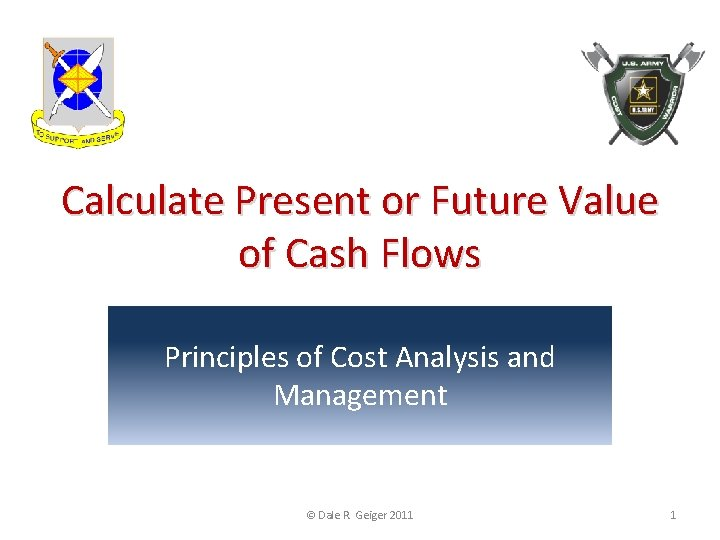Calculate Present or Future Value of Cash Flows Principles of Cost Analysis and Management © Dale R. Geiger 2011 1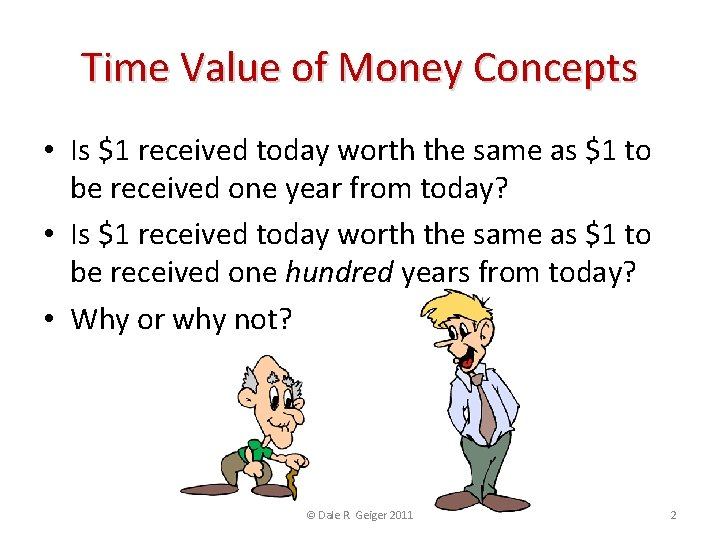Time Value of Money Concepts • Is \$1 received today worth the same as \$1 to be received one year from today? • Is \$1 received today worth the same as \$1 to be received one hundred years from today? • Why or why not? © Dale R. Geiger 2011 2Terminal Learning Objective • Action: Calculate Present Or Future Value Of A Variety Of Cash Flow Scenarios • Condition: You are a cost advisor technician with access to all regulations/course handouts, and awareness of Operational Environment (OE)/Contemporary Operational Environment (COE) variables and actors • Standard: with at least 80% accuracy • Identify and enter relevant report data to solve Present and Future Value equations using macro enabled cash flow templates © Dale R. Geiger 2011 3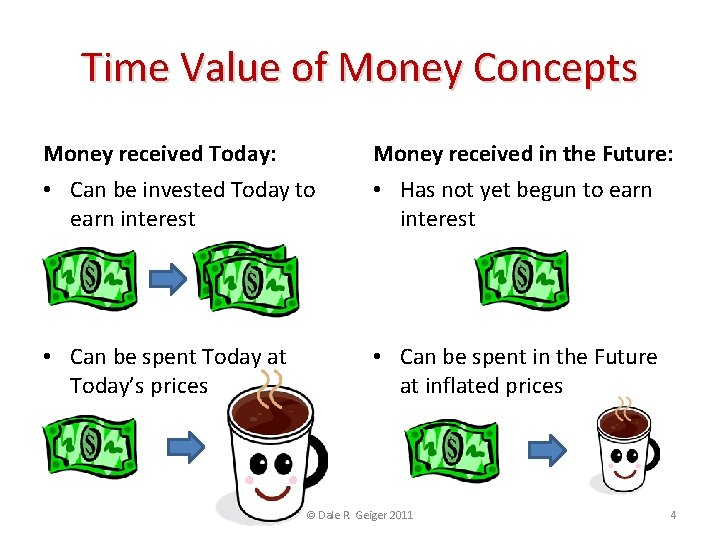Time Value of Money Concepts Money received Today: Money received in the Future: • Can be invested Today to earn interest • Has not yet begun to earn interest • Can be spent Today at Today’s prices • Can be spent in the Future at inflated prices © Dale R. Geiger 2011 4Simple Interest • Interest earned on Principal only Principal * Annual Interest Rate * Time in Years • Invest \$1 today at 10% interest for 3 years Interest = \$1 *. 10 * 3 = \$. 30 • \$1 grows to \$1. 30 over 3 years © Dale R. Geiger 2011 5Compound Interest or Future Value • Invest \$1 today at 10% Interest for 3 years Principal * 10% (1 year) = Interest New Balance \$1. 00 \$1. 10 \$1. 21 *. 10 = \$. 11 = \$. 12 \$1. 10 \$1. 21 \$1. 33 • This relationship can be expressed as: Principal * (1 + Annual Interest Rate)Time in Years \$1*(1+. 10)3 = \$1. 33 © Dale R. Geiger 2011 6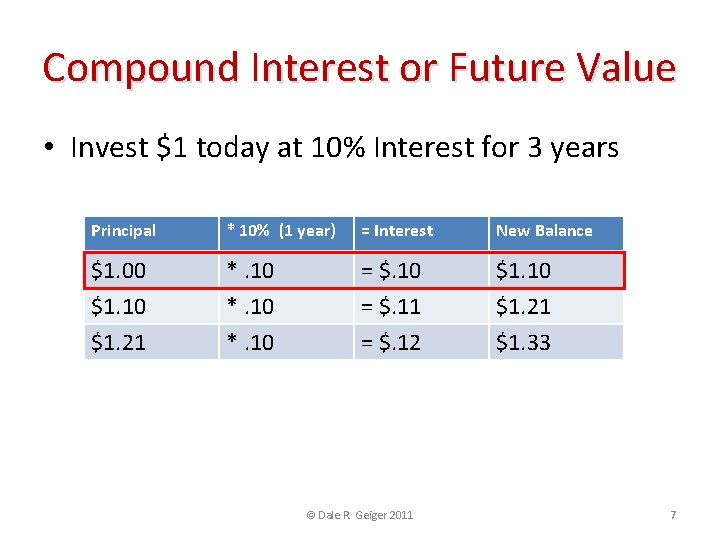Compound Interest or Future Value • Invest \$1 today at 10% Interest for 3 years Principal * 10% (1 year) = Interest New Balance \$1. 00 \$1. 10 \$1. 21 *. 10 = \$. 11 = \$. 12 \$1. 10 \$1. 21 \$1. 33 • This relationship can be expressed as: Principal * (1 + Annual Interest Rate)Time in Years \$1*(1+. 10)3 = \$1. 33 © Dale R. Geiger 2011 7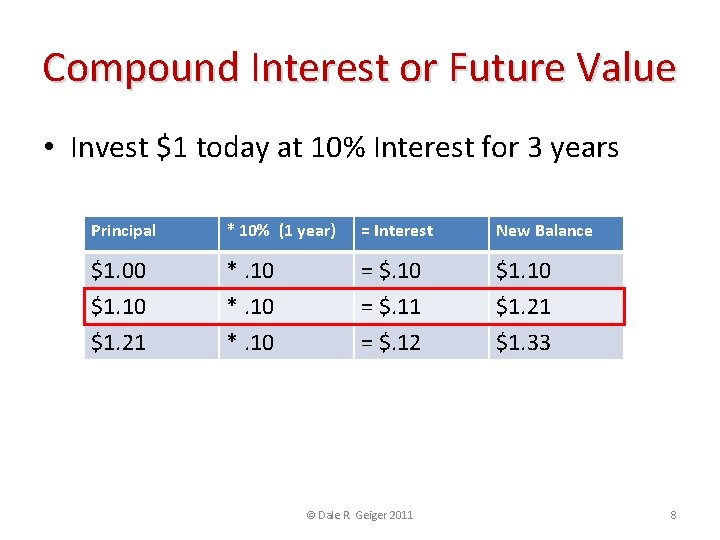Compound Interest or Future Value • Invest \$1 today at 10% Interest for 3 years Principal * 10% (1 year) = Interest New Balance \$1. 00 \$1. 10 \$1. 21 *. 10 = \$. 11 = \$. 12 \$1. 10 \$1. 21 \$1. 33 • This relationship can be expressed as: Principal * (1 + Annual Interest Rate)Time in Years \$1*(1+. 10)3 = \$1. 33 © Dale R. Geiger 2011 8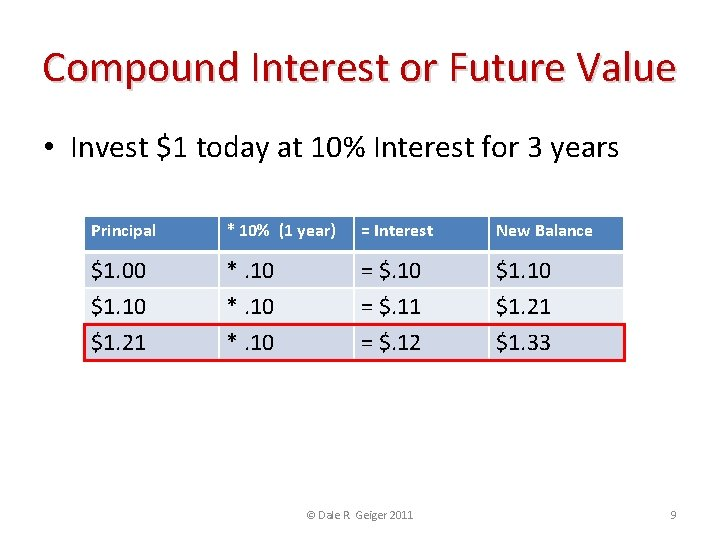Compound Interest or Future Value • Invest \$1 today at 10% Interest for 3 years Principal * 10% (1 year) = Interest New Balance \$1. 00 \$1. 10 \$1. 21 *. 10 = \$. 11 = \$. 12 \$1. 10 \$1. 21 \$1. 33 • This relationship can be expressed as: Principal * (1 + Annual Interest Rate)Time in Years \$1*(1+. 10)3 = \$1. 33 © Dale R. Geiger 2011 9Compound Interest or Future Value • Invest \$1 today at 10% Interest for 3 years Principal * 10% (1 year) = Interest New Balance \$1. 00 \$1. 10 \$1. 21 *. 10 = \$. 11 = \$. 12 \$1. 10 \$1. 21 \$1. 33 • This relationship can be expressed as: Principal * (1 + Annual Interest Rate)Time in Years \$1*(1+. 10)3 = \$1. 33 © Dale R. Geiger 2011 10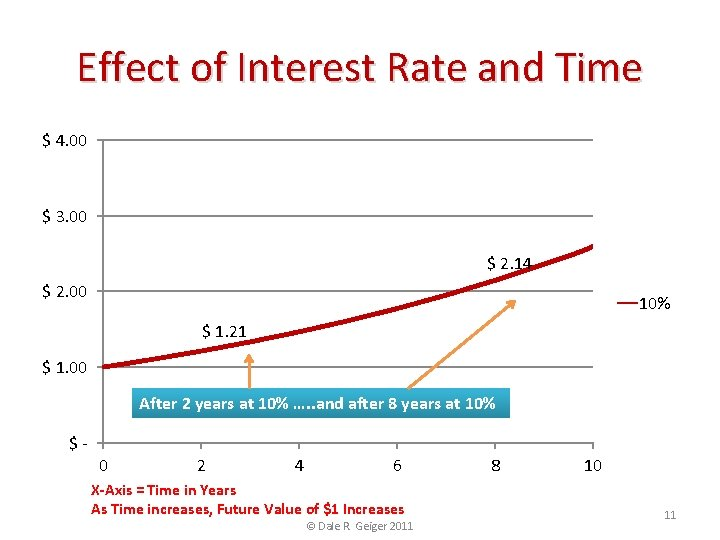Effect of Interest Rate and Time \$ 4. 00 \$ 3. 00 \$ 2. 14 \$ 2. 00 10% \$ 1. 21 \$ 1. 00 After 2 years at 10% …. . and after 8 years at 10% \$- 0 2 4 6 X-Axis = Time in Years As Time increases, Future Value of \$1 Increases © Dale R. Geiger 2011 8 10 11Effect of Interest Rate and Time \$ 4. 00 A higher interest rate causes the future value to increase more in the same 8 years. \$ 3. 00 \$ 3. 06 15% \$ 2. 14 \$ 2. 00 10% 5% \$ 1. 48 \$ 1. 00 \$- 0 2 4 6 X-Axis = Time in Years As interest rate increases, Future Value of \$1 Increases © Dale R. Geiger 2011 8 10 12The Future Value Table The Value of \$1 at 10% interest after 8 years is \$2. 14 The Factors are pre-calculated on the FV Table. © Dale R. Geiger 2011 13Check on Learning • How does compound interest differ from simple interest? • How does number of years affect the future value of an investment? © Dale R. Geiger 2011 14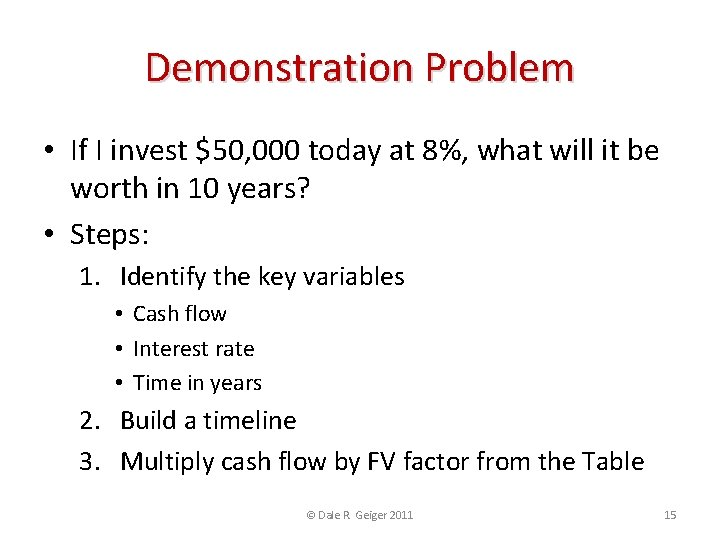Demonstration Problem • If I invest \$50, 000 today at 8%, what will it be worth in 10 years? • Steps: 1. Identify the key variables • Cash flow • Interest rate • Time in years 2. Build a timeline 3. Multiply cash flow by FV factor from the Table © Dale R. Geiger 2011 15Identify Key Variables • Cash Flows • \$50, 000 to be paid now • Cash Payments are negative numbers • Some unknown amount to be received ten years in the future • Cash Receipts are positive numbers • Interest Rate = 8% • Time in Years = 10 © Dale R. Geiger 2011 16Build a Timeline \$ 120 K ? 100 \$50, 000 to be invested now 80 60 Unknown amount to be received in 10 years 40 20 0 -20 0 1 2 3 4 5 6 7 8 9 10 -40 \$ -60 K \$50 K X-Axis = Time in Years © Dale R. Geiger 2011 17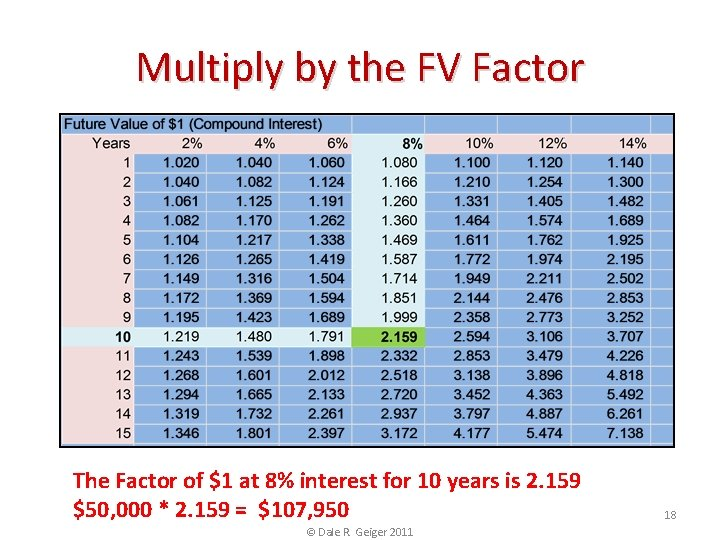Multiply by the FV Factor The Factor of \$1 at 8% interest for 10 years is 2. 159 \$50, 000 * 2. 159 = \$107, 950 © Dale R. Geiger 2011 18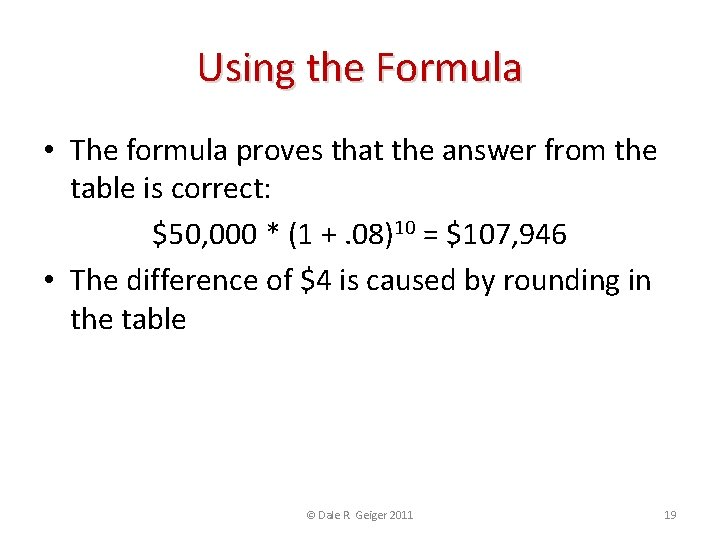Using the Formula • The formula proves that the answer from the table is correct: \$50, 000 * (1 +. 08)10 = \$107, 946 • The difference of \$4 is caused by rounding in the table © Dale R. Geiger 2011 19Proof Year Principal 1 \$50, 000 2 \$54. 000 3 \$58, 320 4 5 6 7 8 9 10 \$62, 986 \$68, 024 \$73, 466 \$79, 343 \$85, 690 \$92, 545 \$99, 949 *8% = Interest *. 08 = \$4, 000 = \$4, 320 = \$4, 666 \$54, 000 \$58, 320 \$62, 986 *. 08 *. 08 = \$5, 039 = \$5, 442 = \$5, 877 = \$6, 347 = \$6, 855 = \$7, 404 = \$7, 996 \$68, 024 \$73, 466 \$79, 343 \$85, 690 \$92, 545 \$99, 949 \$107, 945 © Dale R. Geiger 2011 New Balance 20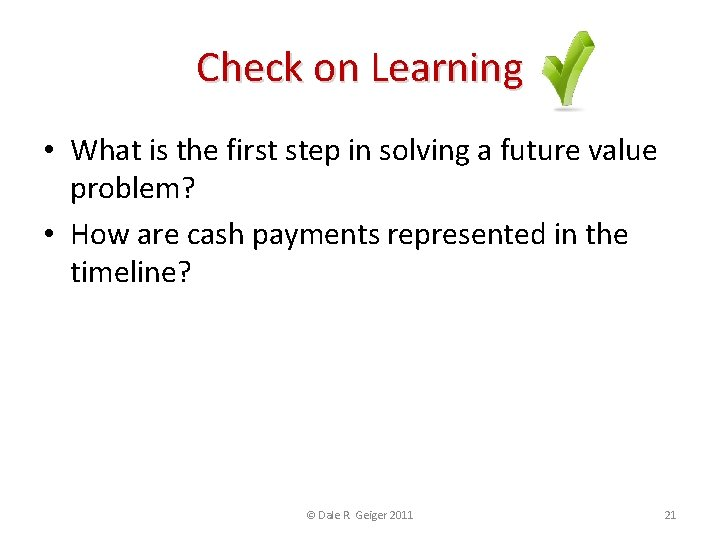Check on Learning • What is the first step in solving a future value problem? • How are cash payments represented in the timeline? © Dale R. Geiger 2011 21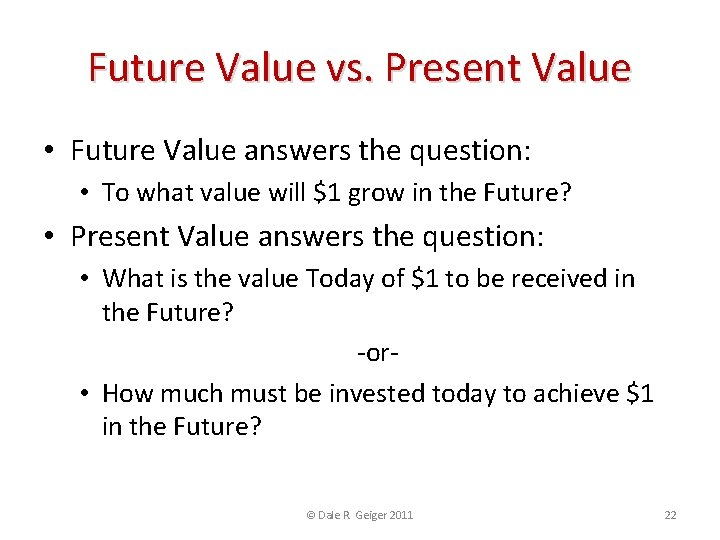Future Value vs. Present Value • Future Value answers the question: • To what value will \$1 grow in the Future? • Present Value answers the question: • What is the value Today of \$1 to be received in the Future? -or • How much must be invested today to achieve \$1 in the Future? © Dale R. Geiger 2011 22Future Value vs. Present Value The value of a dollar received today will increase in the future A dollar to be received in the future is worth less than a dollar received today © Dale R. Geiger 2011 23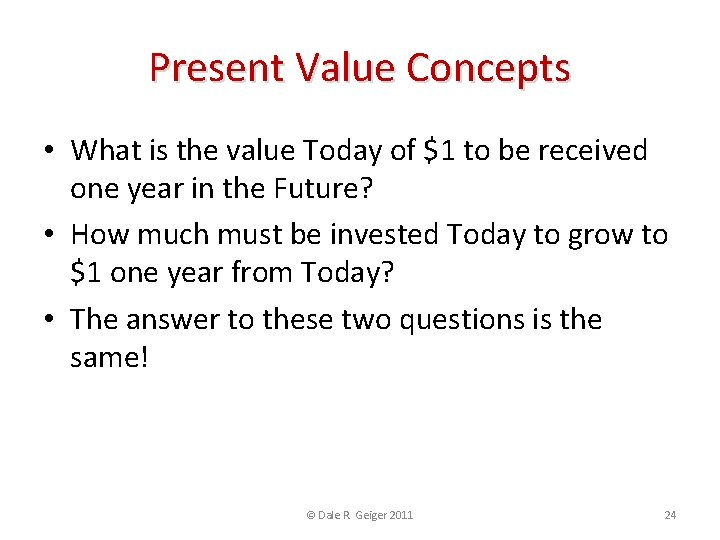Present Value Concepts • What is the value Today of \$1 to be received one year in the Future? • How much must be invested Today to grow to \$1 one year from Today? • The answer to these two questions is the same! © Dale R. Geiger 2011 24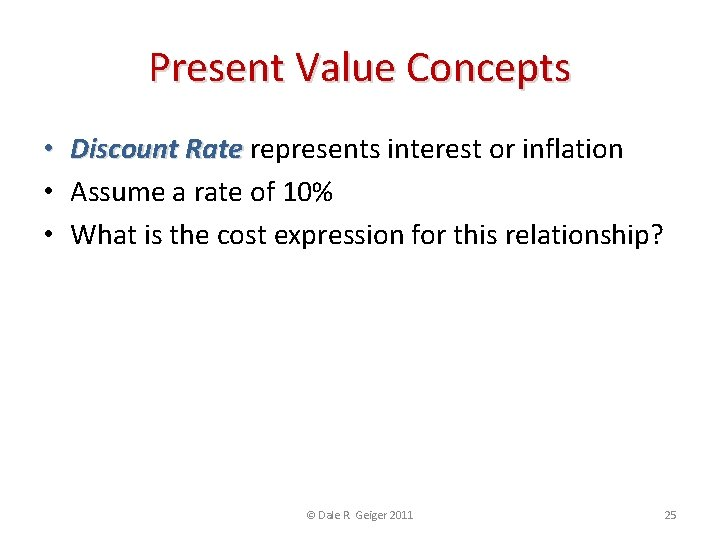Present Value Concepts • Discount Rate represents interest or inflation • Assume a rate of 10% • What is the cost expression for this relationship? \$Investment Today + Interest = \$1. 00 -or\$Investment + (\$Investment *. 10) = \$1. 00 \$Investment * (1+. 10) = \$1. 00 \$Investment = \$1/(1. 10) \$Investment = \$. 91 © Dale R. Geiger 2011 25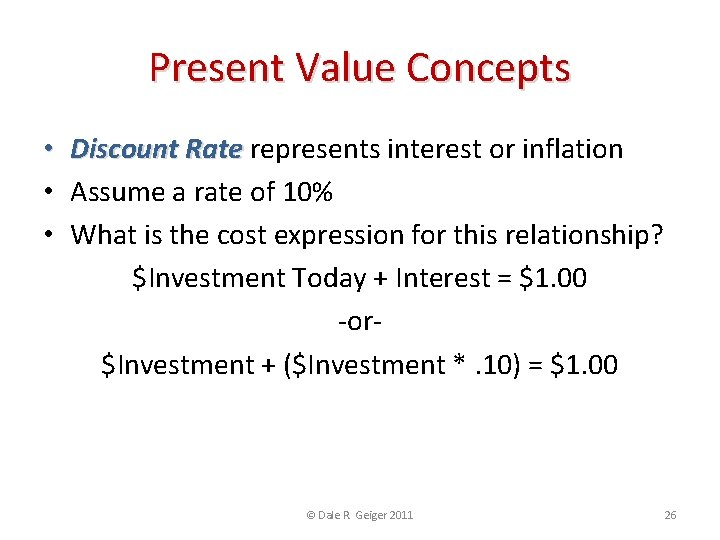Present Value Concepts • Discount Rate represents interest or inflation • Assume a rate of 10% • What is the cost expression for this relationship? \$Investment Today + Interest = \$1. 00 -or\$Investment + (\$Investment *. 10) = \$1. 00 \$Investment * (1+. 10) = \$1. 00 \$Investment = \$1/(1. 10) \$Investment = \$. 91 © Dale R. Geiger 2011 26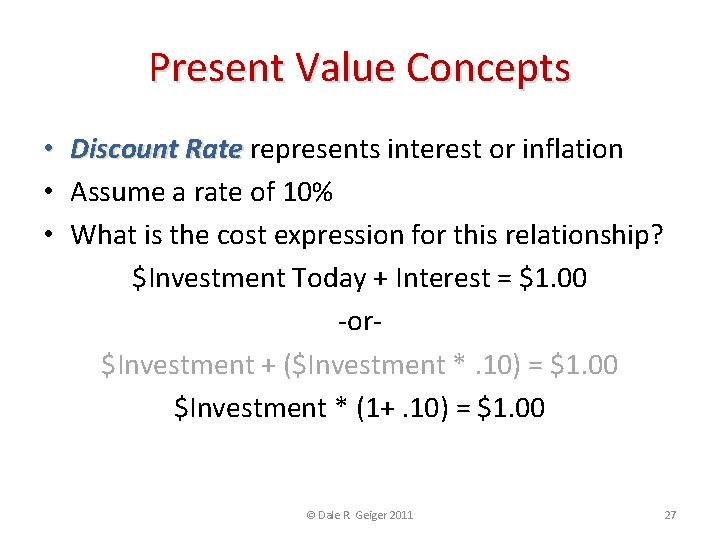Present Value Concepts • Discount Rate represents interest or inflation • Assume a rate of 10% • What is the cost expression for this relationship? \$Investment Today + Interest = \$1. 00 -or\$Investment + (\$Investment *. 10) = \$1. 00 \$Investment * (1+. 10) = \$1. 00 \$Investment = \$1/(1. 10) \$Investment = \$. 91 © Dale R. Geiger 2011 27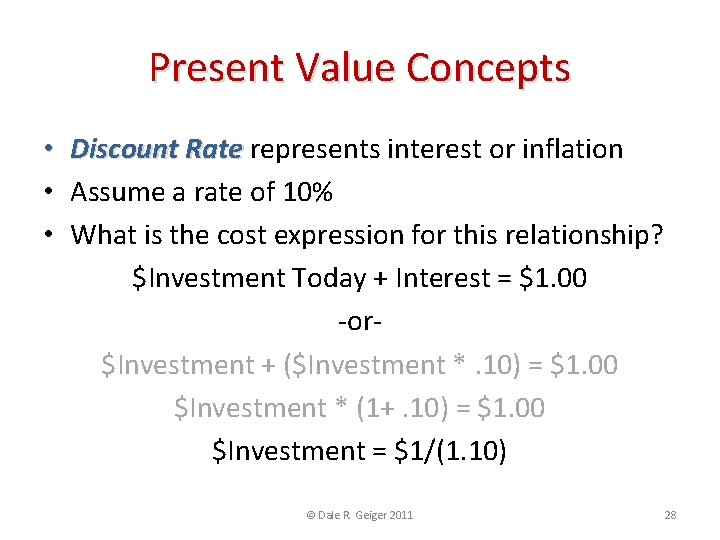Present Value Concepts • Discount Rate represents interest or inflation • Assume a rate of 10% • What is the cost expression for this relationship? \$Investment Today + Interest = \$1. 00 -or\$Investment + (\$Investment *. 10) = \$1. 00 \$Investment * (1+. 10) = \$1. 00 \$Investment = \$1/(1. 10) \$Investment = \$. 91 © Dale R. Geiger 2011 28Present Value Concepts • Discount Rate represents interest or inflation • Assume a rate of 10% • What is the cost expression for this relationship? \$Investment Today + Interest = \$1. 00 -or\$Investment + (\$Investment *. 10) = \$1. 00 \$Investment * (1+. 10) = \$1. 00 \$Investment = \$1/(1. 10) \$Investment = \$. 91 © Dale R. Geiger 2011 29Proof • Plug \$. 91 in to the original equation: \$. 91 + (\$. 91 *. 10) = \$1. 00 \$. 91 +. 09 = \$1. 00 • This relationship is fairly simple for one period, but what about multiple periods? © Dale R. Geiger 2011 30Present Value Concepts • How much must be invested today to achieve \$1. 00 three years from today? • What is the cost expression for this relationship? \$Investment * (1 + Rate) #Years = \$Future Value \$Investment = \$Future Value / (1 + Rate) #Years -or\$Investment * (1+. 10) 3 = \$1. 00 \$Investment = \$1. 00 / (1+. 10) 3 \$Investment = \$. 75 © Dale R. Geiger 2011 31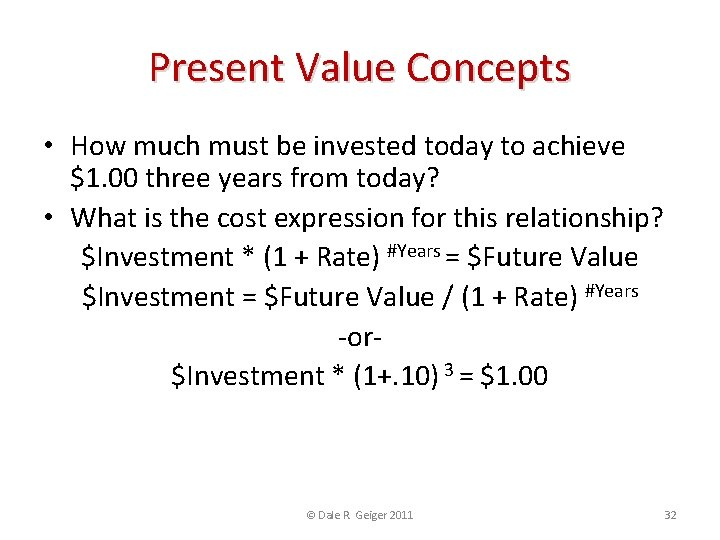Present Value Concepts • How much must be invested today to achieve \$1. 00 three years from today? • What is the cost expression for this relationship? \$Investment * (1 + Rate) #Years = \$Future Value \$Investment = \$Future Value / (1 + Rate) #Years -or\$Investment * (1+. 10) 3 = \$1. 00 \$Investment = \$1. 00 / (1+. 10) 3 \$Investment = \$. 75 © Dale R. Geiger 2011 32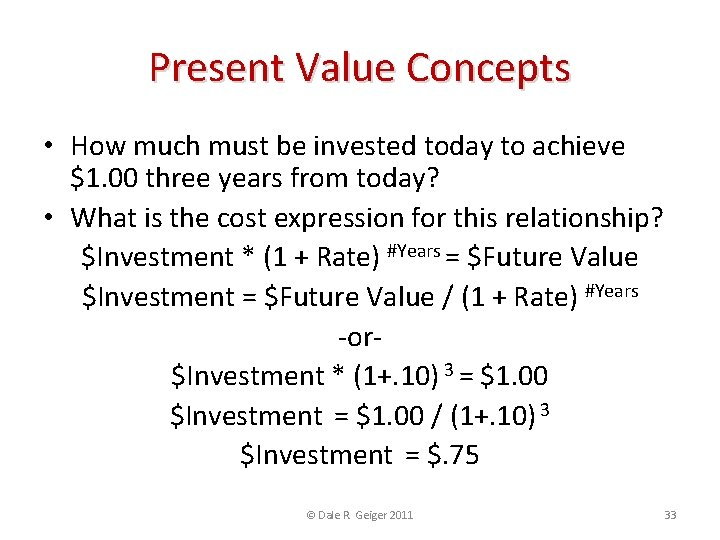Present Value Concepts • How much must be invested today to achieve \$1. 00 three years from today? • What is the cost expression for this relationship? \$Investment * (1 + Rate) #Years = \$Future Value \$Investment = \$Future Value / (1 + Rate) #Years -or\$Investment * (1+. 10) 3 = \$1. 00 \$Investment = \$1. 00 / (1+. 10) 3 \$Investment = \$. 75 © Dale R. Geiger 2011 33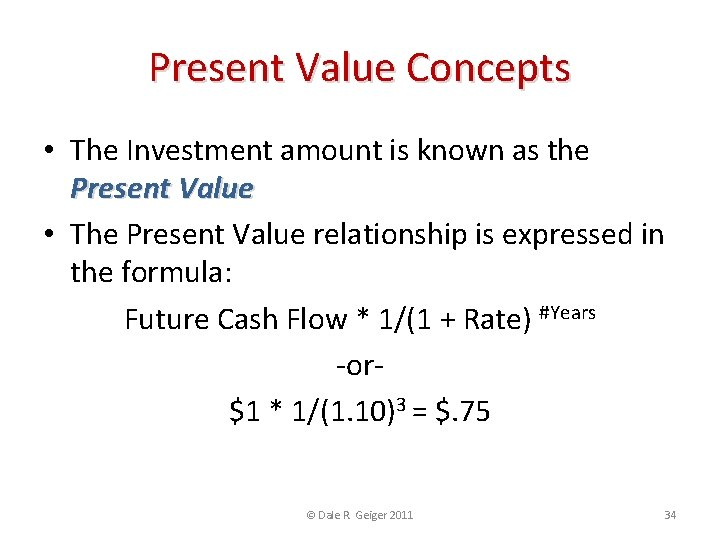Present Value Concepts • The Investment amount is known as the Present Value • The Present Value relationship is expressed in the formula: Future Cash Flow * 1/(1 + Rate) #Years -or\$1 * 1/(1. 10)3 = \$. 75 © Dale R. Geiger 2011 34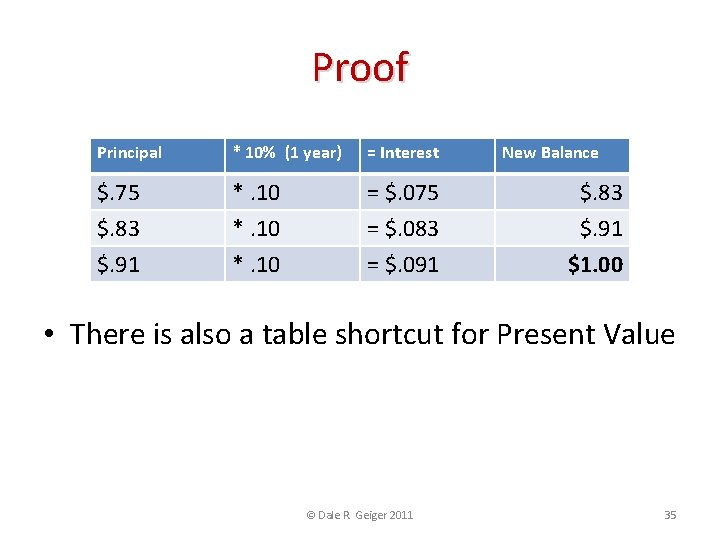Proof Principal * 10% (1 year) = Interest \$. 75 \$. 83 \$. 91 *. 10 = \$. 075 = \$. 083 = \$. 091 New Balance \$. 83 \$. 91 \$1. 00 • There is also a table shortcut for Present Value © Dale R. Geiger 2011 35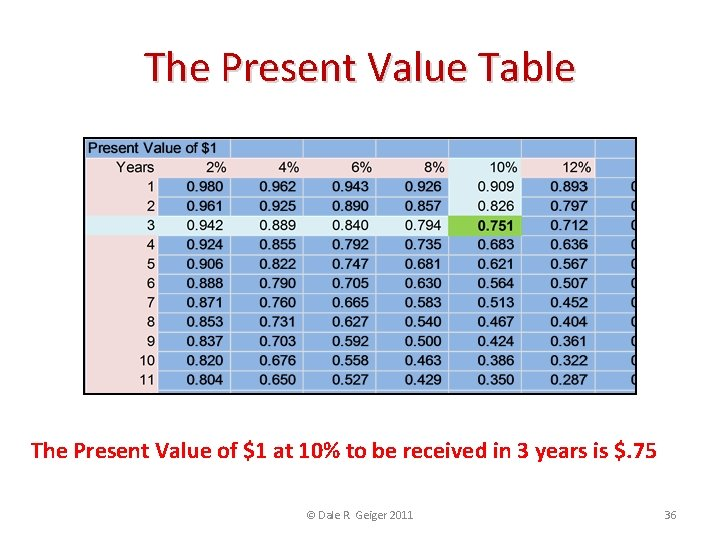The Present Value Table The Present Value of \$1 at 10% to be received in 3 years is \$. 75 © Dale R. Geiger 2011 36Effect of Interest Rate and Time \$ 1. 20 \$ 1. 00 \$ 0. 83 \$ 0. 80 \$ 0. 60 10% \$ 0. 47 \$ 0. 40 \$ 0. 20 \$- \$1 to be received in 2 years at 10% …. . and in 8 years at 10% 0 2 4 6 X-Axis = Time in Years As Time increases, Present Value of \$1 Decreases © Dale R. Geiger 2011 8 10 37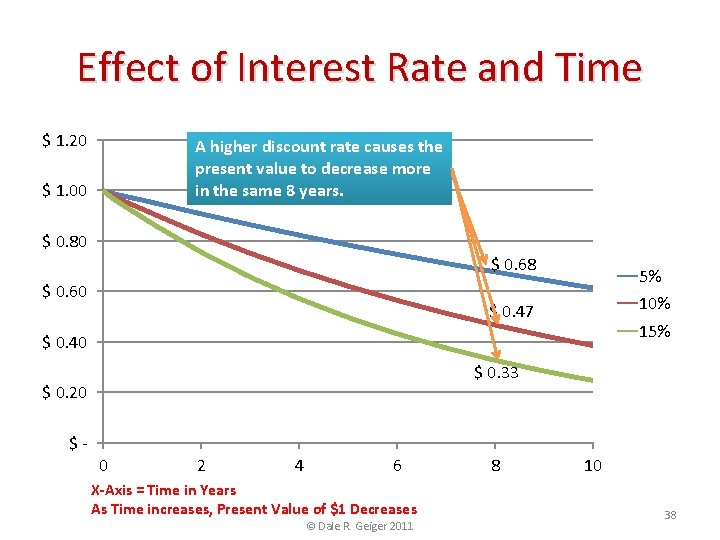Effect of Interest Rate and Time \$ 1. 20 A higher discount rate causes the present value to decrease more in the same 8 years. \$ 1. 00 \$ 0. 80 \$ 0. 68 \$ 0. 60 5% 10% \$ 0. 47 15% \$ 0. 40 \$ 0. 33 \$ 0. 20 \$- 0 2 4 6 X-Axis = Time in Years As Time increases, Present Value of \$1 Decreases © Dale R. Geiger 2011 8 10 38Check on Learning • What does Present Value represent? • How does the Present Value table differ from the Future Value table? © Dale R. Geiger 2011 39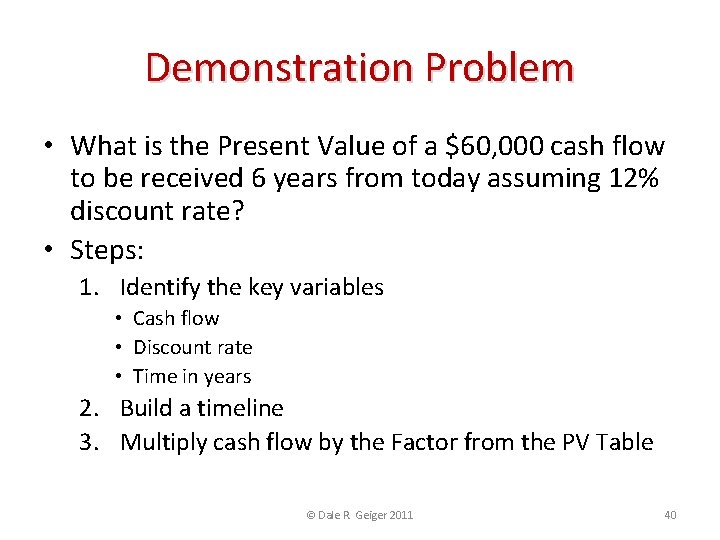Demonstration Problem • What is the Present Value of a \$60, 000 cash flow to be received 6 years from today assuming 12% discount rate? • Steps: 1. Identify the key variables • Cash flow • Discount rate • Time in years 2. Build a timeline 3. Multiply cash flow by the Factor from the PV Table © Dale R. Geiger 2011 40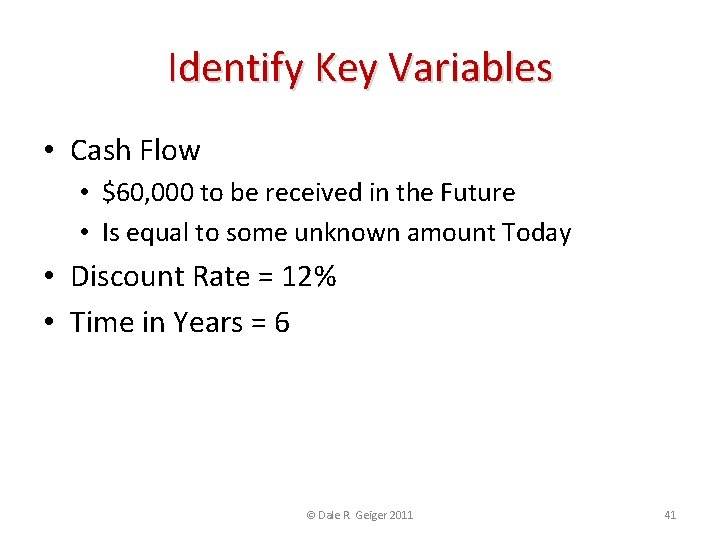Identify Key Variables • Cash Flow • \$60, 000 to be received in the Future • Is equal to some unknown amount Today • Discount Rate = 12% • Time in Years = 6 © Dale R. Geiger 2011 41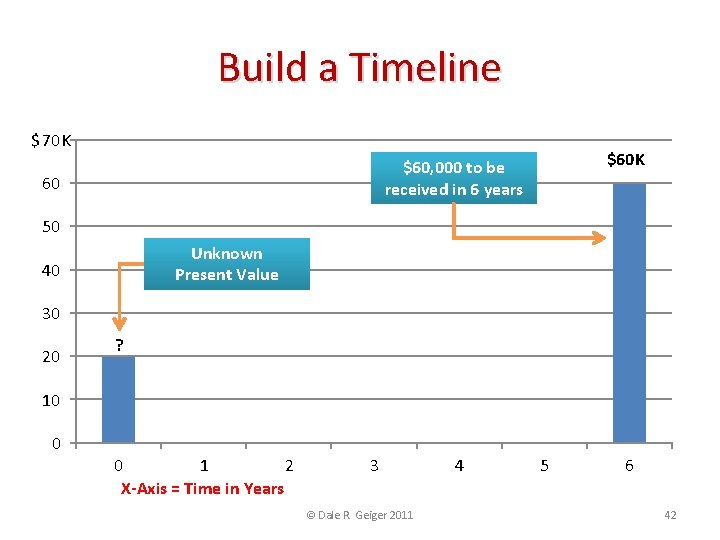Build a Timeline \$ 70 K \$60, 000 to be received in 6 years 60 50 Unknown Present Value 40 30 20 ? 10 0 0 1 2 X-Axis = Time in Years 3 © Dale R. Geiger 2011 4 5 6 42Multiply by the PV Factor The Factor of \$1 at 12% discount for 6 years is 0. 507 \$60, 000 * 0. 507 = \$30, 420 © Dale R. Geiger 2011 43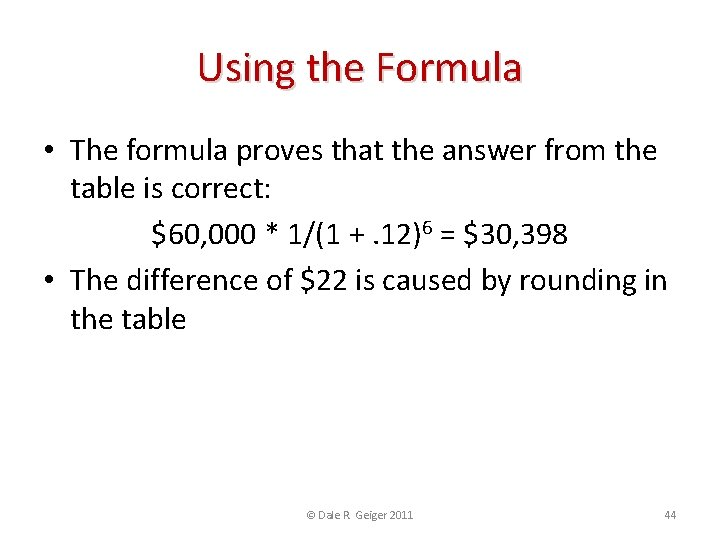Using the Formula • The formula proves that the answer from the table is correct: \$60, 000 * 1/(1 +. 12)6 = \$30, 398 • The difference of \$22 is caused by rounding in the table © Dale R. Geiger 2011 44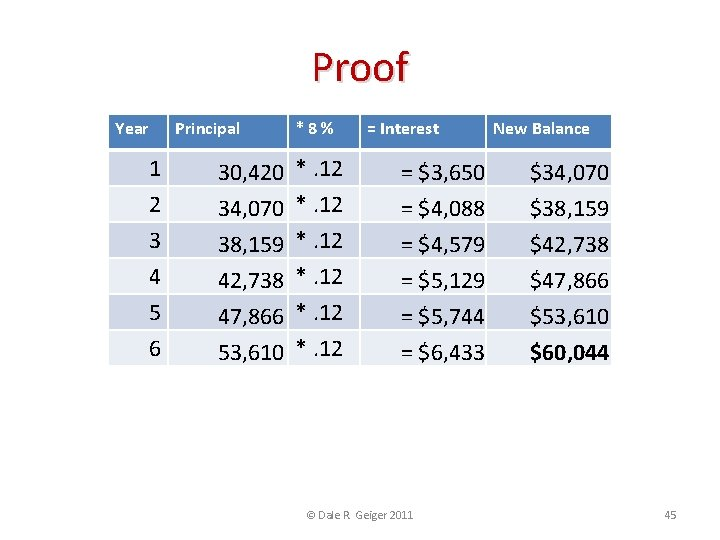Proof Year 1 2 3 4 5 6 Principal 30, 420 34, 070 38, 159 42, 738 47, 866 53, 610 *8% *. 12 = Interest = \$3, 650 = \$4, 088 = \$4, 579 = \$5, 129 = \$5, 744 = \$6, 433 © Dale R. Geiger 2011 New Balance \$34, 070 \$38, 159 \$42, 738 \$47, 866 \$53, 610 \$60, 044 45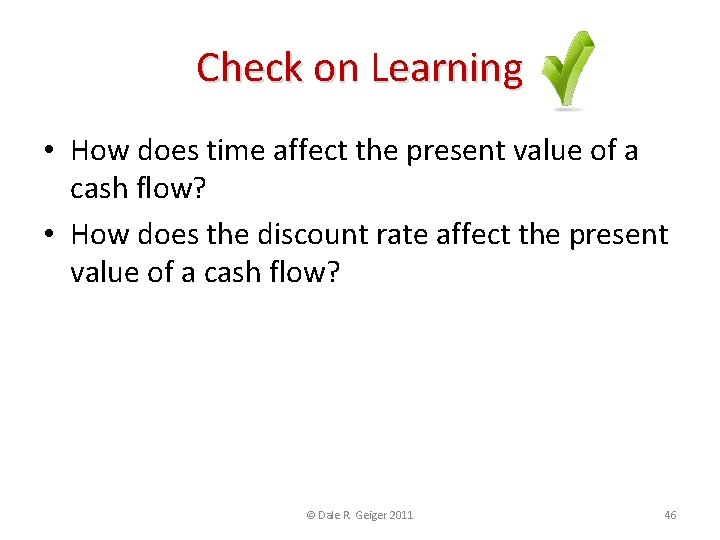Check on Learning • How does time affect the present value of a cash flow? • How does the discount rate affect the present value of a cash flow? © Dale R. Geiger 2011 46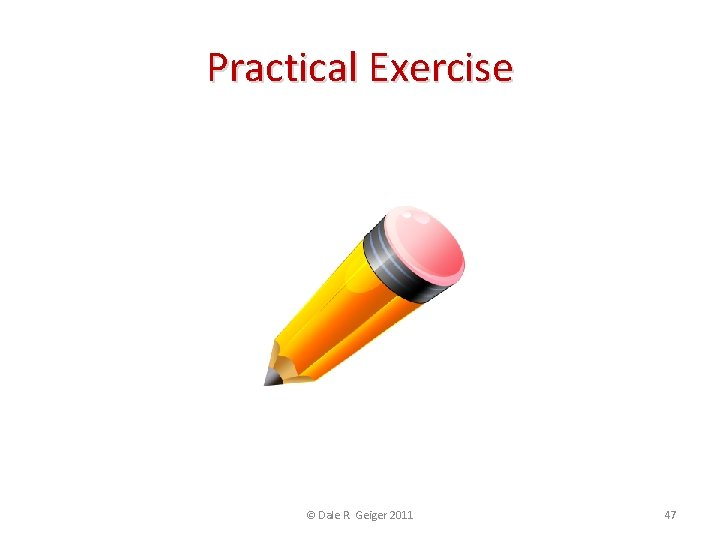Practical Exercise © Dale R. Geiger 2011 47Time Value of Money Worksheet Enter key variables in the blank white cells to generate the graph shown below © Dale R. Geiger 2011 48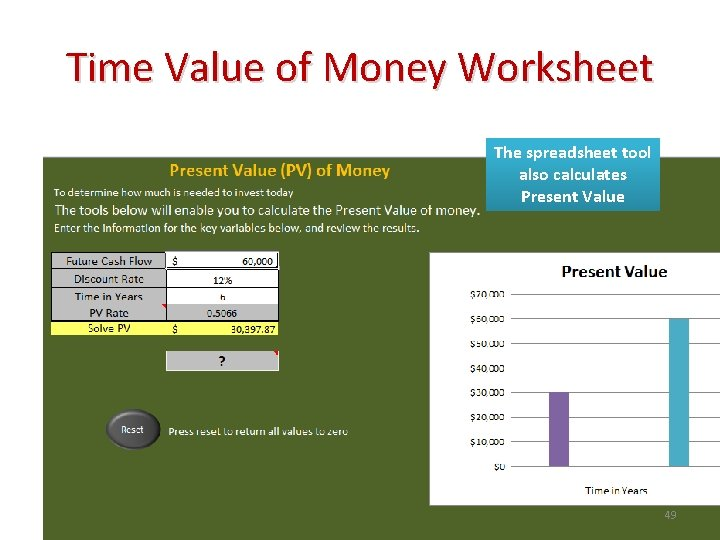Time Value of Money Worksheet The spreadsheet tool also calculates Present Value © Dale R. Geiger 2011 49Practical Exercise © Dale R. Geiger 2011 50